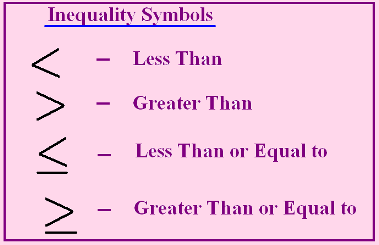# Inequalities

Inequalities are a way to express the relative size of two numbers or objects, that being that one of those objects is smaller, greater or equal in size in relation to the other object. You can express inequalities graphically via a number line or as a mathematical expression. A number line is a straight line on which every point represents a single real number and it is very easy to portrait inequalities on it. For example, let us say you get an expression that says:

X is greater than 9.

The solutions of this problem (or the value of X) are values on the number line that are greater than 9 and you should mark these values. Be careful not to mark the number 9 as well, because the assignment asks only for the values greater than that number. Now, let us say that the assignment states that:

X is greater than or equal to 9

In this case the solution would include the number 9 because it is permitted in the text of the assignment that x can be equal to 9.

Inequalities also come in forms of longer mathematical expressions, similar to equations. Depending on the number of steps you have to perform in order to solve them, they can also be divided into one-step inequalities, two-step inequalities or multi-step inequalities.

Inequalities can be in five different ways and each of them represents different relations between the objects that are being compared (levitra online). We are going to explain them to you in this table using both mathematical symbols and text.

 Symbols Meaning a < b The value of a is less than the value of b a > b The value of a is greater than the value of b a ≠ b The value of a is not equal to the value of b a ≤ b The value of a is less than or equal to the value of b a ≥ b The value of a is greater than or equal to the value of b

The expression for the value of a being not equal to the value of b can also be expressed with the symbol “<>” instead of the symbol “≠”, but they mean the same. In this case, a can be any number on the number line except b.## Properties of inequalities

Inequalities have five basic properties: transitivity, addition and subtraction, multiplication and division, additive inverse and finally, multiplicative inverse.

The property of transitivity  states that for a, b, and c being any real numbers:

 If a < b and b > c then a > c If a > b and b < c then a < c If a ≤ b and b = c then a > c If a ≥ b and b = c then a < c

The rules that deal with the properties of addition and subtraction within inequalities say that for a, b and c being any real numbers:

 If a < b then a + c < b + c and a – c < b – c If a > b then a + c > b + c and a – c > b – c

The rules that deal with the properties of multiplication and division within inequalities state that for a, b and c being any real numbers:

 If c positive and a < b then ac < bc and a/c < b/c If c negative and a < b then ac > bc and a/c > b/c

The property of the additive inverse says that for a and b being any real numbers:

 If a < b then – a > – b If a > b then – a < – b

The property of the multiplicative inverse states has two parts. If a and b are both positive or both negative real numbers, then:

 If a < b then 1/a > 1/b If a > b then 1/a < 1/b

If either a or b is negative, then:

 If a < b then 1/a < 1/b If a > b then 1/a > 1/b

If you wish to practice with inequalities, check the free worksheets below.

## Inequalities exams for teachers

 Exam Name File Size Downloads Upload date Integers Graph inequalities – Integers – easy 245.3 kB 7668 September 3, 2019 Graph inequalities – Integers – medium 234.6 kB 10514 September 3, 2019 Graph inequalities – Integers – hard 238.7 kB 4679 September 3, 2019 Decimals Graph inequalities – Decimals – easy 223.2 kB 3031 September 3, 2019 Graph inequalities – Decimals – medium 221 kB 3045 September 3, 2019 Graph inequalities – Decimals – hard 224.6 kB 3029 September 3, 2019 Fractions Graph inequalities – Fractions – easy 232.4 kB 2439 September 3, 2019 Graph inequalities – Fractions – medium 243.1 kB 2369 September 3, 2019 Graph inequalities – Fractions – hard 270 kB 2159 September 3, 2019

## Inequalities worksheets for students

 Worksheet Name File Size Downloads Upload date Graph inequalities – Integers 2.4 MB 6294 September 3, 2019 Graph inequalities – Decimals 2.2 MB 2979 September 3, 2019 Graph inequalities – Fractions 746.5 kB 3057 September 3, 2019 Inequality for given graph 527.1 kB 4320 September 3, 2019